# Part 6: Planar Transformations

In this guide, we explain what translations, reflections and rotations of multiple of 90˚ are with detailed diagrams. We'll also give you some checkpoint questions to test your knowledge and solutions.Planar transformations produce interesting patterns which can be useful in everyday life.

For example, mirrors reflect objects allowing cars to see around corners, and roundabouts rotate cars allowing them to pass through intersections.

If we are provided with a coordinate system such as the cartesian plane, we can study these transformations in mathematical detail.

## NSW Syllabus Outcomes

 Stage 4 NSW Syllabus Syllabus Explanation Describe translations, reflections in an axis, and rotations of multiples of $$90°$$ on the Cartesian plane using coordinates (ACMMG181) Use the notation to name the ‘image‘ resulting from a transformation of a point on the Cartesian planePlot and determine the coordinates for resulting from translating one or more timesPlot and determine the coordinates for resulting from reflecting in either the $$x-axis$$ or $$y-axis$$Plot and determine the coordinates for resulting from rotating by a multiple of $$90°$$ about the originRecognise that a combination of translations and/or reflections and/or rotations can produce the same result as another combination of translations and/or reflections and/or rotations

## Assumed knowledge for Planar transformations

Students should have developed spatial awareness and understand how to plot points on a cartesian plane.

## Planar transformation

The three types of planar transformations covered are translations, rotations and reflections.

Translating points means moving them along the plane by a certain, consistent amount.

For example, the following shape and its image are a translation: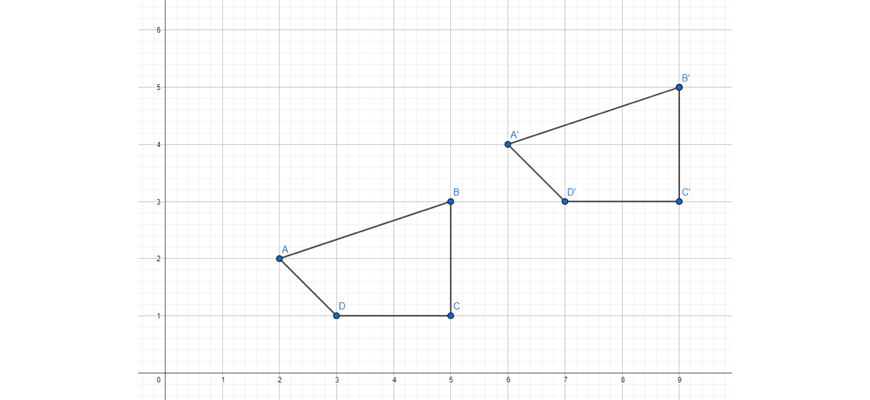To determine a mathematical rule for a translation, we should consider corresponding points on the original shape and its image.

Here, we can consider $$A$$ and $$A’$$:

• $$\ A$$ is $$(2,2)$$ and $$A’$$ is $$(6,4)$$.

We can see that the $$x-coordinate$$ has changed from $$2$$ to $$6$$ and the $$y-coordinate$$ has changed from $$2$$ to $$4$$.

So we have translated $$4$$ across and $$2$$ up.

Hence, the rule for this translation is $$A(x,y) \rightarrow A'(x + 4, \ y + 2)$$

Using this translation, if we are given an original point such as $$B(5,3)$$, we can find $$B’$$ by applying the same translation:

• $$B’$$ is $$(5+4,3+2)$$ which is $$(9,5)$$.

Rotating points involves moving the points by an angle relative to a given point.

For example, the following shape has been rotated about $$(0,0)$$ by $$90$$ degrees anticlockwise: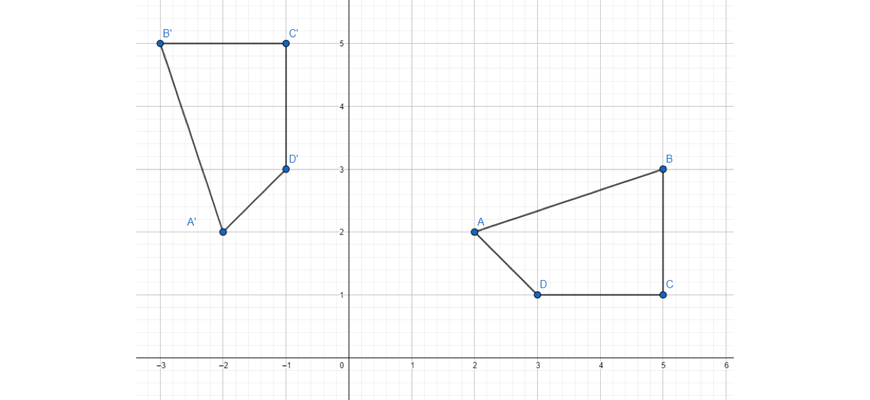Again, to find the mathematical rule, let’s consider the coordinates of corresponding points.

From $$B(5,3)$$ to $$B’(-3,5)$$, we have swapped $$x$$ with $$-y$$ and $$y$$ with $$x$$.

So we can express our rule as $$(x,y) \rightarrow (-y,x)$$.

Using this planar transformation, we can find the coordinates of another point, such as $$C(5,1)$$, whose image will be $$C(5,1) \rightarrow C’ (-1,5)$$.

Reflecting points involves copying the points across a line so that their distance to the line is preserved.

For example, the following shape has been reflected about the $$x-axis$$: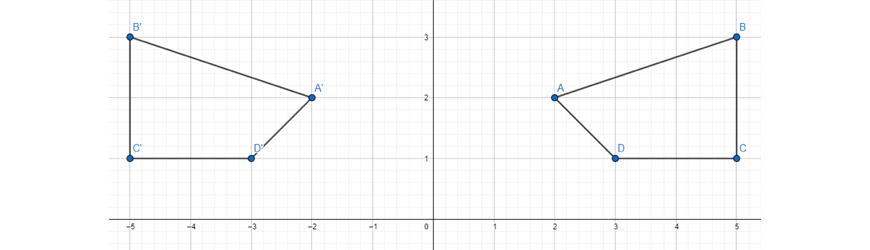To determine a mathematical rule for a reflection, we should consider corresponding points on the original shape and its image.

If we consider $$C$$ and $$C’$$:

• $$C$$ is $$(5,1)$$ and $$C’$$ is $$(-5,1)$$.

We can see that the $$x-coordinate$$ has changed from $$5$$ to $$-1$$ and the $$y-coordinate$$ remains unchanged.

Hence we can express the rule for this translation as $$C(x,y) \rightarrow C'(-x,y)$$.

Using this transformation, we can find the coordinates of other points, such as $$D(3,1) \rightarrow D'(-3,1)$$.

## Checkpoint Questions

1. Translate the following set of points $$3$$ units up and $$2$$ units left. Then, determine a mathematical rule for the given translation.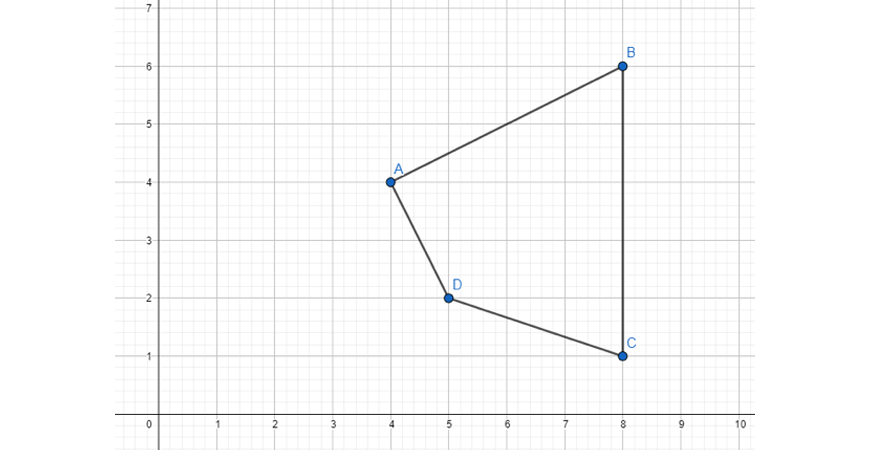2. Rotate the following set of points by $$90$$ degrees clockwise around the origin. Then, determine a mathematical rule for the given translation.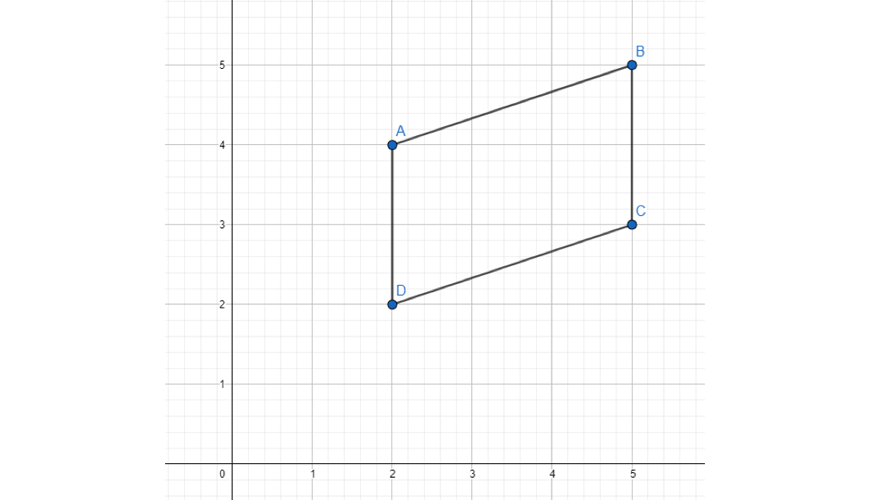3. Reflect the following set of points across the $$y-axis$$. Then, determine a mathematical rule for the given translation.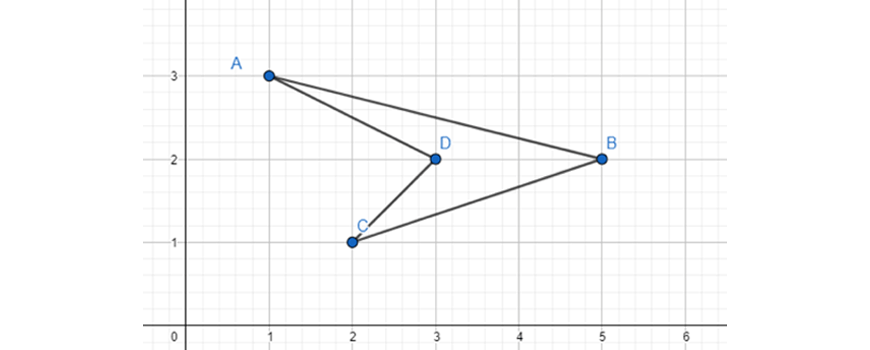## Solutions

1. $$P(x,y) \rightarrow P'(x-2, \ y+3)$$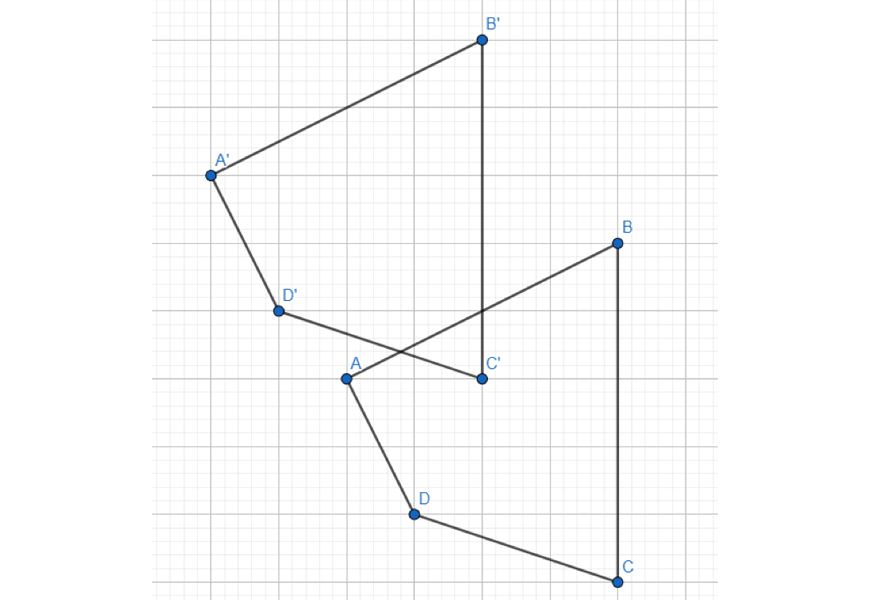2. $$P(x,y) \rightarrow P'(y, -x)$$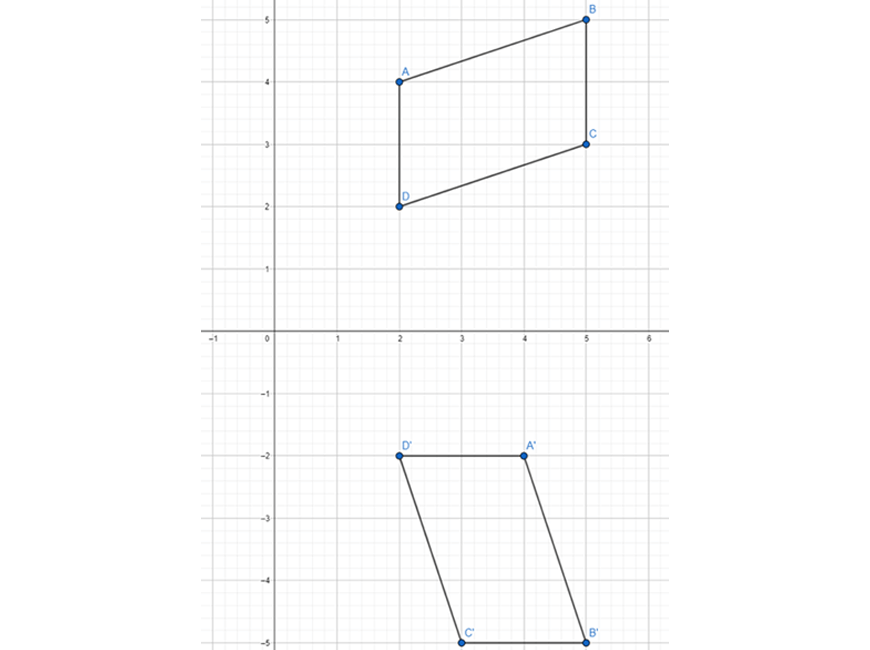3. $$P(x,y) \rightarrow P(x, -y)$$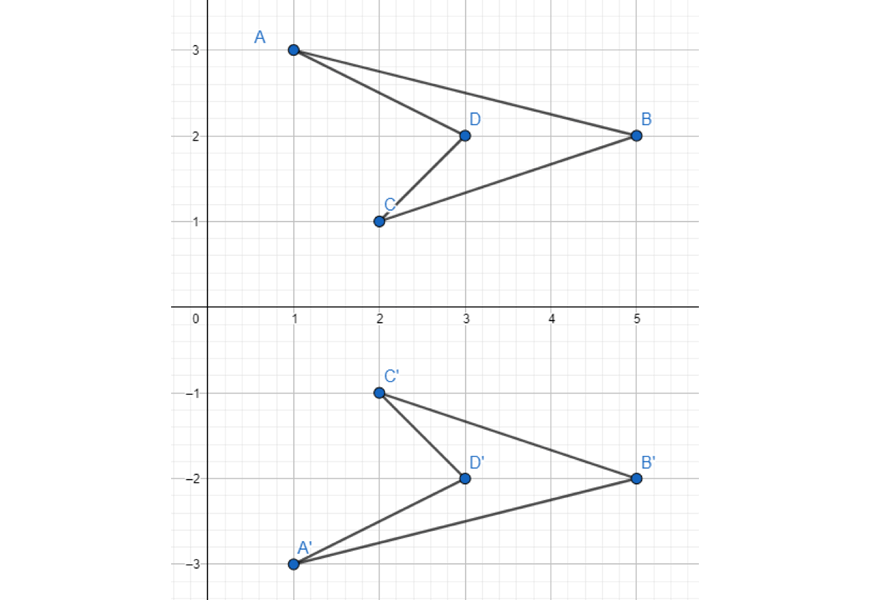## Summary of Planar Transformations

Translation, rotation and reflection are three common planar translations of objects on a coordinate plane.

In this article, we demonstrated how you can define mathematical rules for each of these transformations to apply them to different points.

© Matrix Education and www.matrix.edu.au, 2021. Unauthorised use and/or duplication of this material without express and written permission from this site’s author and/or owner is strictly prohibited. Excerpts and links may be used, provided that full and clear credit is given to Matrix Education and www.matrix.edu.au with appropriate and specific direction to the original content.